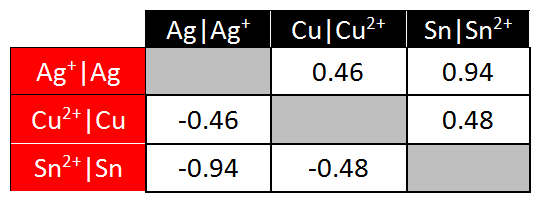# Electrochemistry

-Introduction

Step 1:

-Investigating redox reactions

-Practice with redox reactions

-Reduction tendencies of metal ions

Step 2:

-Electron transfer

-Electrochemical cell

-Practice with cells

-Powering a stopwatch

Step 3:

-Measuring potentials

-Calculating potentials

-Practice with potentials

-Applying potentials

Step 4:

-Non-standard conditions

-Practice with non-standard cells

Feedback Form:

Electrochemistry Tutorial: Galvanic Cells and the Nernst Equation >> Step 3: Calculating cell potentials

 < Previous | Next >

# Electrochemistry: Galvanic Cells and the Nernst Equation

## Step 3: Calculating cell potentials

In the preceding simulations you measured cell potentials of several cells made of various combinations of half-cells. It would be a monumental task to assemble a list of all possible cells and report a cell voltage for each. It is more useful to create a list of the potentials of half-cells and then calculate the potentials of the required combinations. Here, we will first learn how to use half-cell potentials, and then consider how to determine half-cell potentials from experiments.

The voltage of an electrochemical cell depends on the redox reaction occurring in the cell. Above, we saw that it is often useful to break a redox reaction, such as:

Zn(s) + Pb2+(aq) --> Zn2+(aq) + Pb(s)

into the following half reactions.

 Half-reaction Process Half-cell location Pb2+(aq) + 2 e- --> Pb(s) Reduction Cathode (electron sink) Zn(s) --> Zn2+(aq) + 2 e- Oxidation Anode (electron source)

Given how useful half-cell potentials are (you can use them to calculate the potential of any full-cell), it is not surprising that these are well tabulated in handbooks and on the web. These tables, by convention, contain the half-cell potentials for reduction. Reduction potential, the tendency to gain electrons, represents the relative tendency for a given metal ion to undergo reduction. For the above cell, the table would contain:

 Pb2+(aq) + 2 e- --> Pb(s) Eº = -0.13V Zn2+(aq) + 2 e- --> Zn(s) Eº = -0.76V

The Pb reaction in the table and in the cell are in the same direction (i.e. reduction). This will always be the case for the cathode, because the table lists reduction potentials and reduction always occurs at the cathode. However, the Zn reaction is in the opposite direction. This will always be the case for the anode, because the table lists reduction potentials and oxidation occurs at the anode. Because of this, the cell potential is the difference between the cell potential of the cathode and that of the anode:

Eºcell = Eºcathode - Eºanode

The only potential we can measure experimentally is that of a full cell (a reduction process must always be coupled with an oxidation process, otherwise free electrons would build up). So all measurements correspond to differences between the numbers in a table of half-cell potentials. This is analogous to the altitudes of cities. All we really care about is the difference in altitudes between cities. All tables define altitude relative to sea level, but if we instead created a table that gave the altitudes of cities relative to the top of Mt Everest (or the center of the earth), we would still get the same values for the difference in altitudes between cities. Sea level is the "reference" for altitude, meaning that sea level has an altitude of zero.

For half-cell potentials, we also need to decide on a "reference". The convention among chemists is to take the following half-reaction as the standard and assign it a zero half-cell potential:

 2 H+(aq) + 2 e- --> H2(g) Eº = 0.00V

The electrode corresponding to this half-reaction is called the "standard hydrogen electrode". Here the notation Eº is used to represent hydrogen's standard electrode potential (declared to be zero at all temperatures). The measured cell voltage using the standard hydrogen electrode as one of the half-cells is, therefore, the potential of the other half reaction. The standard hydrogen electrode consists of hydrogen gas at 1 atmosphere and so is not convenient for us to work with in the laboratory. Instead, you will create your own table of reduction potentials using a tin half-cell as a standard instead of the hydrogen half-cell.

Using the information from a standard reduction potentials table (see link below), the standard cell potential for the zinc/lead cell is then:

Eºcell = Eºcathode - Eºanode = -0.13 - (- 0.76) = 0.63 V

The following example shows how to use a table of standard reduction potentials [such as the one available online at: http://hyperphysics.phy-astr.gsu.edu/hbase/tables/electpot.html] to predict the cell potentials that you measured in the simulation on the previous page:For the reaction between Cu and Sn, we can perform the following calculation:

 Cu2+(aq) + 2 e- --> Cu(s) Eºcathode = 0.34 (half-cell potential) Sn(s) --> Sn2+(aq) + 2 e- Eºanode = - 0.14 (half-cell potential)

Since the copper half-cell is the cathode (this is where the reduction occurs) and the tin half-cell is the anode (where the oxidation occurs), our calculation would be:

Eºcell = Eºcathode - Eºanode = 0.34 - (- 0.14) = 0.48 V

For the reaction between Cu and Ag, we can perform the following calculation:

 2 * (Ag+(aq) + 1 e- --> Ag(s)) Eºcathode = 0.80 (half-cell potential) Cu(s) --> Cu2+(aq) + 2 e- Eºanode = 0.34 (half-cell potential)

Since the silver half-cell is the cathode (this is where the reduction occurs) and the copper half-cell is the anode (where the oxidation occurs), our calculation would be:

Eºcell = Eºcathode - Eºanode = 0.80 - 0.34 = 0.46 V

Note that we multiplied the Ag half-cell reaction by 2, to balance the electrons. However, we did not multiply the cell potential by 2. When we multiple a half-cell reaction by a constant, we do not need to alter the half-cell potential.

 < Previous | Next >